Next: 7.4 Thermal Analysis of Up: 7. Simulation Results Previous: 7.2 Five-Stage CMOS Ring

# 7.3 Five-Stage CML Ring Oscillator

Most of the bipolar logic families such as T2L and I2L are saturated-mode logic circuits. As the transistors are driven into saturation, circuit speed is diminished. Several techniques have been considered to prevent saturation such as Schottky clamping. To prevent the transistors from saturating, the current and voltage swings have to be limited. The operation of non-saturating logic is based on current switching and is hence known as current mode logic (CML). A CML gate is an emitter coupled logic (ECL) gate stripped of the emitter-follower  which provided the power gain for driving external circuits. Also the gate propagation delay is increased by the transit time of the transistor, the overall speed is more than compensated by the reduced RC time constant that the external load would have placed on the switching pair . However, due to the improved fabrication technologies the situation is considerably different from that in which ECL was developed and the emitter-follower can be considered an unnecessary artifact of the past.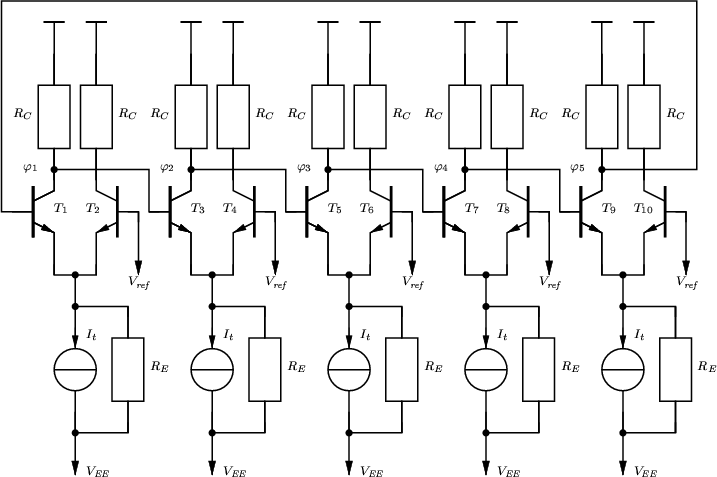The DC transfer characteristic of a single stage without load from Fig. 6.12 can be approximated by assuming a simple Ebers-Moll model for the transistors [71,1]. The input voltage is compared to the reference voltage

 Vin =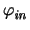- Vref (7.8) = VBE1 - VBE2 (7.9) = VT .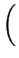ln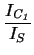- ln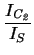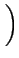= VT .ln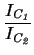. (7.10)

Neglecting the base currents and RE, the sum of the collector currents must be equal to the current flowing through the current source, hence

 IC1 + IC2 = It   . (7.11)

With the voltage drops at RC bothand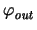can be calculated as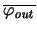= - IC1 . RC = -(7.12)= - IC2 . RC = -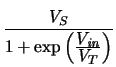(7.13)

with

 VS = It . RC (7.14)

The voltage gain of the single inverter is

 Av =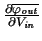= -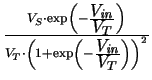(7.15)

with a maximum at Vin = 0 V

 Avmax = -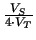(7.16)

For Avmax greater than unity VS must be greater than 4 . VT. The larger gain is used by the system to account for static and dynamic voltage drops that occur in practice. In practice, a minimum gain of 4 is needed to provide sufficient noise margins [52,74].

For the simulation VS = 20 . VT has been assumed hence a gain of -5 could be expected. When considering an inverter chain consisting of 5 CML inverters as shown in Fig. 7.14 the total gain occurring at the last output node is (- 5)5 = 3125. With such a high gain, the circuit is too sensitive to the voltage changes occurring during iteration so no solution can be found without a proper initial-guess using conventional techniques. However, using the shunt conductance technique with= 4 a DC operating point can be easily obtained with only 34 iterations.

First, starting from the operating point obtained above, the DC open-loop transfer characteristic was determined which is shown in Fig. 7.15. The voltage gain of the circuit is shown in Fig. 7.16 which corresponds approximately to the simple results obtained above.

As there is no unique operating point for the closed-loop one of the node voltages had to be fixed to force the circuit into an initial state from which oscillations can start. Hence, the input voltage of the first inverter was fixed to 0 V during the operating point calculation.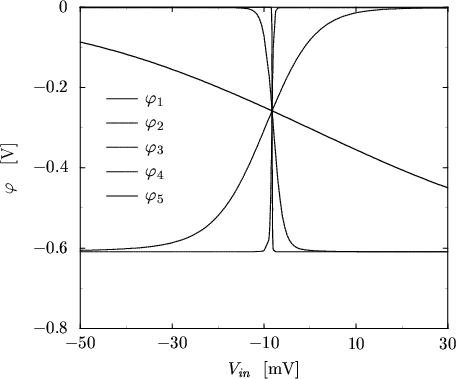Oscillations start immediately with a frequency fDD = 6.8 GHz for DD and fHD = 10.6 GHz for HD which gives a relative difference of 36% for the DD model (Fig. 7.17). This is due to the velocity overshoot which occurs in the base-collector space charge region which cannot be modeled using a DD transport model. The current levels are approximately equal in both cases as shown in Fig. 7.18.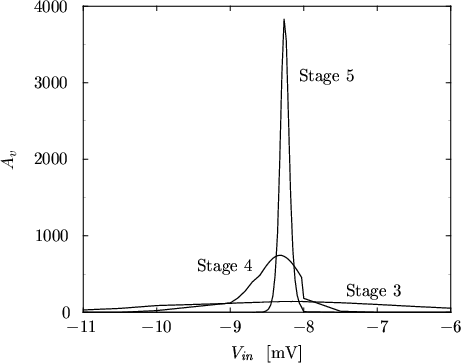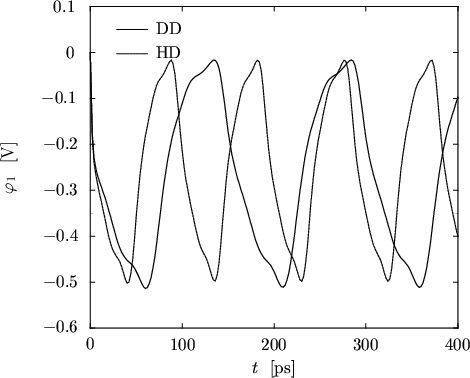Next: 7.4 Thermal Analysis of Up: 7. Simulation Results Previous: 7.2 Five-Stage CMOS Ring
Tibor Grasser
1999-05-31